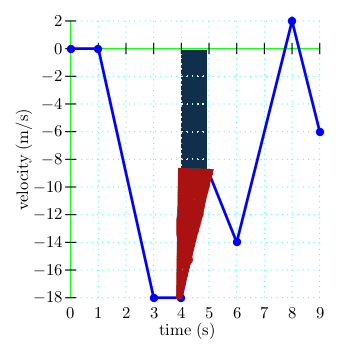# Distance From a Velocity-Time Graph

I need to know how to determine distance from a velocity time graph. The graph lines are all linear with changes in direction and slope, but no curves.

I've tried finding the area under the lines but that doesn't seem to be it...

I need to know how to determine distance from a velocity time graph. The graph lines are all linear with changes in direction and slope, but no curves.

I've tried finding the area under the lines but that doesn't seem to be it...

Velocity=distance/ time. multiply by the time stamp at whatever point you are on on the graph.

You know that Velocity is a change in position over change in time, correct?

Using that, you should be able to figure out the position. (At least, that's how I do it.)

What did you do when you tried finding the area under the graph?

completing each time increment as a triangle and using 1/2b*h.

completing each time increment as a triangle and using 1/2b*h.

Could you post a picture of the graph?

maybe, lemme try

well uh no actually. idk how D: could i get an e-mail? i think i could e-mail it

it's #7 i need help with!

The blue graph right?

What ares did you use 1/2b * h on (what time)?

Last edited:
i posted a pdf of the question sheet. you can see the graph on the second page. i'm fairly sure i found the area correctly, but wouldn't that give me the displacement? and i need distance... look at number 7 on the pdf. plz :/

What did you find for displacement?

i got -54

Okay, and for what parts did you use 1/2b*h?

because from times 1-3 seconds the line goes from 0 to -18, i did 1/2 the base (y axis time) plus the height of -18. this got -36.
time interval 3-4 seconds have a constant velocity of -18 m/s so i added -18 to the first -18 getting -36. then i found the are from 4-5 by multiplying 1/2 the base of 1 second, by the height of -9, getting an additonal -4.5. Sum of all these? -54!

i meant times the height! sorry i'm a confusing typer !

please ignore the first time i said "this got -36" its a typo...

because from times 1-3 seconds the line goes from 0 to -18, i did 1/2 the base (y axis time) plus the height of -18. this got -36.
time interval 3-4 seconds have a constant velocity of -18 m/s so i added -18 to the first -18 getting -36. then i found the are from 4-5 by multiplying 1/2 the base of 1 second, by the height of -9, getting an additonal -4.5. Sum of all these? -54!

You found the area for time 4-5s wrong.You found the red part, but not the blue part. The blue part is a rectangle.

Also, I think for this problem you can assume displacement and distance are meant to be the same thing.

Last edited: